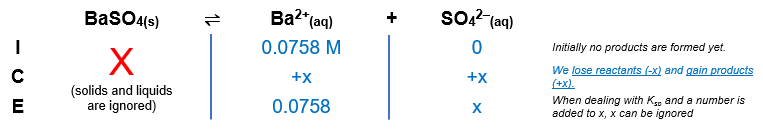# Problem: Calculate the concentration of sulfate ion when BaSO4 just begins to precipitate from a solution that is 0.0758 M in Ba2+.

###### FREE Expert Solution

BaSO4(s)  Ba2+(aq) + SO42–(aq)The Ksp expression for BaSO4 is:

${\mathbf{K}}_{\mathbf{sp}}\mathbf{=}\mathbf{\left[}{\mathbf{Ba}}^{\mathbf{2}\mathbf{+}}\mathbf{\right]}\mathbf{\left[}{{\mathbf{SO}}_{\mathbf{4}}}^{\mathbf{2}\mathbf{-}}\mathbf{\right]}\phantom{\rule{0ex}{0ex}}\frac{\mathbf{1}\mathbf{.}\mathbf{1}\mathbf{×}{\mathbf{10}}^{\mathbf{-}\mathbf{10}}}{\mathbf{0}\mathbf{.}\mathbf{0758}}\mathbf{=}\frac{\mathbf{\left(}\overline{)\mathbf{0}\mathbf{.}\mathbf{0758}}\mathbf{\right)}\mathbf{\left(}\mathbf{x}\mathbf{\right)}}{\overline{)\mathbf{0}\mathbf{.}\mathbf{0758}}}$

99% (483 ratings)###### Problem Details

Calculate the concentration of sulfate ion when BaSO4 just begins to precipitate from a solution that is 0.0758 M in Ba2+.

Frequently Asked Questions

What scientific concept do you need to know in order to solve this problem?

Our tutors have indicated that to solve this problem you will need to apply the Ksp concept. You can view video lessons to learn Ksp. Or if you need more Ksp practice, you can also practice Ksp practice problems.

What textbook is this problem found in?

Our data indicates that this problem or a close variation was asked in Chemistry - OpenStax 2015th Edition. You can also practice Chemistry - OpenStax 2015th Edition practice problems.